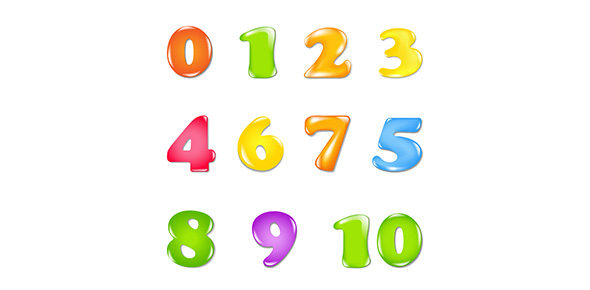# Exam 1 Target 1 Preap Numbers 1-5

Approved & Edited by ProProfs Editorial Team
At ProProfs Quizzes, our dedicated in-house team of experts takes pride in their work. With a sharp eye for detail, they meticulously review each quiz. This ensures that every quiz, taken by over 100 million users, meets our standards of accuracy, clarity, and engagement.
| Written by Sfduke
S
Sfduke
Community Contributor
Quizzes Created: 57 | Total Attempts: 8,166
Questions: 5 | Attempts: 100Settings.

• 1.

### Which of the following situations represents a positive displacement of a carton? Assume positive position is measured vertically upward along a y-axis.

• A.

A. A delivery person waiting for an elevator lowers a carton onto a dolly.

• B.

B. When the elevator doors open, the delivery person lifts the dolly over the threshold of the elevator.

• C.

C. The delivery person pushes the dolly to the back of the elevator while pressing a floor button.

• D.

D. The door closes and the elevator moves from the 10th to the 4th floors.

B. B. When the elevator doors open, the delivery person lifts the dolly over the threshold of the elevator.
Explanation
In this situation, the delivery person is lifting the carton vertically upward, which represents a positive displacement along the y-axis.

Rate this question:

• 2.

### What is the correct description of any change of position farther to the right of zero?

• A.

A. positive displacement

• B.

B. positive distance

• C.

C. positive position

• D.

D. positive change of displacement

A. A. positive displacement
Explanation
The correct description of any change of position farther to the right of zero is positive displacement. Displacement refers to the change in position of an object, and when the change is farther to the right of zero, it indicates a positive displacement.

Rate this question:

• 3.

### A dog walks from +4 m to +2 m. Which of the following statements is true about the dog’s motion?

• A.

A. xi = +2 m

• B.

B. xf = +2 m

• C.

C. delta x = +2 m

• D.

D. vavg = 2 m/s

B. B. xf = +2 m
Explanation
The correct answer is b. xf = +2 m. This statement is true because xf represents the final position of the dog, and in this case, the dog's final position is +2 m.

Rate this question:

• 4.

### Rank in decreasing order the displacements of objects having the following pairs of average velocity and time of motion.I. vavg = +2.0 m/s, Dt = 2.0 sII. vavg = +3.0 m/s, Dt = 2.0 sIII. vavg = -3.0 m/s, Dt = 3.0 s

• A.

A. I, II, III

• B.

B. II, III, I

• C.

C. II, I, III

• D.

D. III, II, I

A. A. I, II, III
Explanation
The correct answer is a. I, II, III. This is because the displacement of an object is directly proportional to the product of its average velocity and the time of motion. Therefore, the object with the highest average velocity and shortest time of motion (I) will have the highest displacement, followed by the object with the second highest average velocity and second shortest time of motion (II), and finally the object with the lowest average velocity and longest time of motion (III).

Rate this question:

• 5.

### What is the maximum negative displacement a dog could have if it started its motion at +3 m?

• A.

A. +7 m

• B.

B. +3 m

• C.

C. -3 m

• D.

D. -7 mBack to top